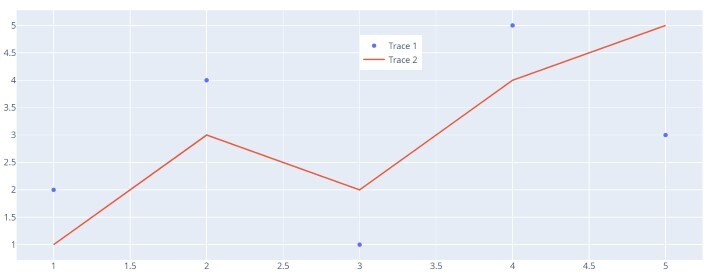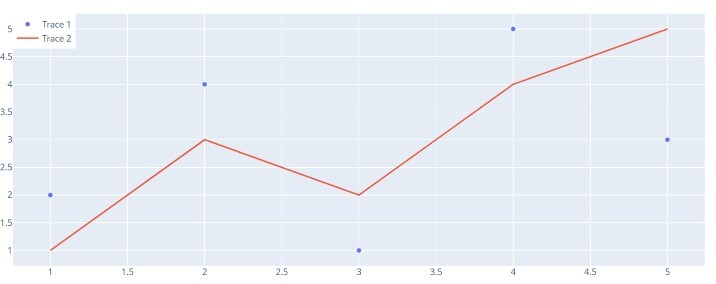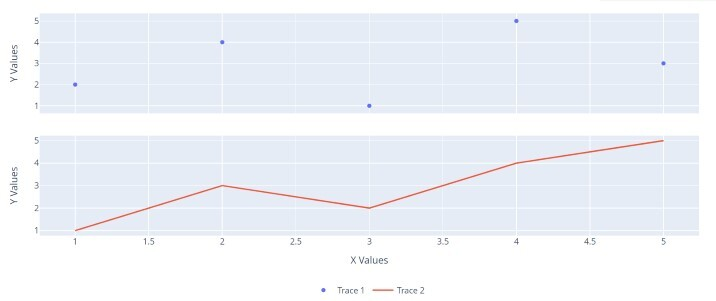# 如何在Plotly-Python中将图例定位在图表内部？

## 使用layout.legend属性

### 示例：

import plotly.graph_objects as go
# Test information
x_values = [1, 2, 3, 4, 5]
y_values1 = [2, 4, 1, 5, 3]
y_values2 = [1, 3, 2, 4, 5]
# Create traces
trace1 = go.Scatter(x=x_values, y=y_values1, name='Trace 1', mode='markers')
trace2 = go.Scatter(x=x_values, y=y_values2, name='Trace 2', mode='lines')
# Create the figure
fig = go.Figure([trace1, trace2])
# Update the design to put the legend inside the plot
fig.update_layout(legend=dict(x=0.5, y=0.9))  # Change x and y facilitates on a case-by-case basis
# Show the plot
fig.show()


### 输出## 自动调整大小的图例定位

### 示例

import plotly.graph_objects as go

# Test information
x_values = [1, 2, 3, 4, 5]
y_values1 = [2, 4, 1, 5, 3]
y_values2 = [1, 3, 2, 4, 5]

# Create traces
trace1 = go.Scatter(x=x_values, y=y_values1, name='Trace 1', mode='markers')
trace2 = go.Scatter(x=x_values, y=y_values2, name='Trace 2', mode='lines')

# Create the figure
fig = go.Figure([trace1, trace2])

# Update the design for auto-measuring legend position and left-top anchor
fig.update_layout(legend=dict(x=0, y=1, xanchor='left', yanchor='top'))

# Show the plot
fig.show()


### 输出## 子图中的图例

### 示例

import plotly.graph_objects as go
from plotly.subplots import make_subplots

# Test information
x_values = [1, 2, 3, 4, 5]
y_values1 = [2, 4, 1, 5, 3]
y_values2 = [1, 3, 2, 4, 5]

# Make subplots with two rows and one column
fig = make_subplots(rows=2, cols=1, shared_xaxes=True, vertical_spacing=0.1)

# Add traces to the subplots
fig.add_trace(go.Scatter(x=x_values, y=y_values1, name='Trace 1', mode='markers'), row=1, col=1)
fig.add_trace(go.Scatter(x=x_values, y=y_values2, name='Trace 2', mode='lines'), row=2, col=1)

# Update the design to put legends inside subplots and align them horizontally
fig.update_layout(
legend=dict(x=0.5, y=-0.2, xanchor='center', yanchor='top'), # Adjust y to move legend inside subplot
legend_orientation='h'
)

# Update axis titles
fig.update_xaxes(title_text='X Values', row=2, col=1)
fig.update_yaxes(title_text='Y Values', row=1, col=1)
fig.update_yaxes(title_text='Y Values', row=2, col=1)

# Show the plot
fig.show()


### 输出• 微信订阅
• 回顶